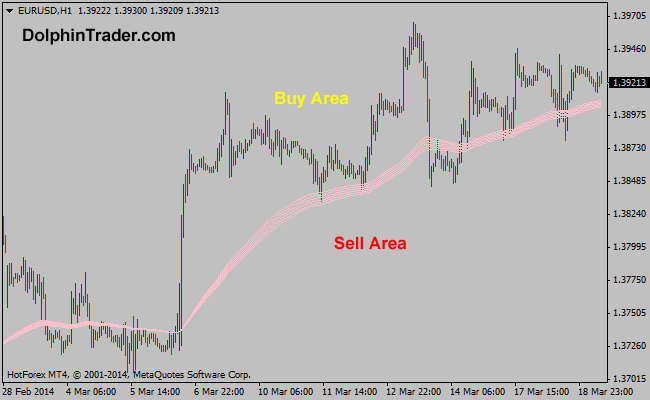# 94 EMA – 114 EMA Guppy Multi Moving Average Metatrader 4 Indicator

This is the fourth GMMA trend trading indicator in a series of 11 indicators entirely based on the Guppy multi moving average. The RainbowMMA_04 consists of the following moving averages: 114 period exponential moving average, 110 period exponential moving average, 106 period exponential moving average, 102 period exponential moving average, 98 period exponential moving average and 94 period exponential moving average.

Buy: Wait for the price to rise above the RainbowMMA_04 GMMA
Sell: Wait for the price to drop below the RainbowMMA_04 GMMA

Indicator Preferences

Currency pairs: any
Time frames: any
Sessions: any